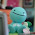## Thursday, January 13, 2011

### Final Percent Post

What is Percent?
A percent is a number out of 100.
ex. 35% is like 35 out of a hundred.

There is another way you can use percent by sing decimal and percent.
ex. 35 i like 0.35 and 35 out of a hundred.

Key Points of this Chapter..

4.1 Represent Percent
To represent a percent, you have to shade squares on a 100 grid, that is called a hundred grid. When you shade in the whole grid that is 100%. To represent greater than 100% shade more than one grid and then. To represent a fractional percent, you have to shade in between 0% and 1%.

4.2 Fractions, Decimal and Percents
When using fractions, decimals and fractions you can use them for representing numbers. You can use percents as a decimal and a fraction.

4.3 Percent of a Number
If you want to find the percent of a number, you have to put the percent as a decimal then you multiply by the number. There are different kind of ways to do this, one way is to divide by 10.

4.4 Combining Percents
When you add the percents together, you can find some problems. To calculate the increase in a number, you can combine percents amount.

Hope you like it (:

#### 1 comment:

1.Great Job Glenn, I liked how yours was very simple. It wasn't too dramatic but it wasn't too dufficult. Great Job, one thing that I would help you with is to add another colour so it doesn't look so boring! Other than that, Great job!